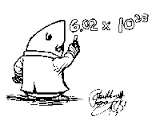Topic 4, Moles & Stoichiometry
Topic 4,
Moles & Stoichiometry
(Key concepts)The concept of the mole:
*One mole of anything = 6 x 10 23rd of those "things"
*Just as one dozen of anything = 12 of those "things"
*6 x 10 23rd is called Avogadro's number
*Used to count atoms, molecules, ions, electrons, etc.
*Two moles equals 12 x 10 23rd particles
*One-half mole equals 3 x 10 23rd particles

Molar mass:
*Molar mass is the mass of one mole
*Molar mass is determined by looking up the atomic mass/masses on the periodic table and using the unit gram
*Examples:
*One mole of Na has a mass of 23 g/mol
*One mole of Cl has a mass of 35 g/mol
*One mole of NaCl has a mass of 58 g/mol
*To find the mass of one mole of Ca(OH)2:
40 + 2(16) + 2(1) = 74 g/mol

Molar volume:
*The volume of one mole of any gas at STP occupies a volume of 22.4 liters
*2 moles of a gas at STP would occupy 44.8 L; 1/2 mole of any gas at STP would occupy 11.2 L

Mole calculations:
*Listed on Table T
*# moles = given mass/molar mass
*Example:
*How many moles in 29 grams of NaCl?
*Solution:  The molar mass of NaCl is 58 g/mol; 29 g/58 g/mol = 0.5 mole

Percent composition by mass:
*Listed on Table T
*% comp = mass of part/mass of whole x 100
*Example:
*What is the percent Na in NaCl?  23/58 x 100 = 40%

Percent hydration:
*Use the percent comp formula
*water (hydration)/whole x 100
*Example:
*What is the percent hydration of
BaCl2-2H2O?
*Solution:  2H2O/BaCl2-2H2O; 2(18)/137 + 2(35) + 2(18) x 100 = 36/243 x 100 = 15%

Stoichiometry:*Must maintain the molar ratios in an equation
*Example:
*2H2  +  O2  -->  2H2O*If use 2 moles of O2, must use 4 moles of H2 and will produce 4 moles of H2O